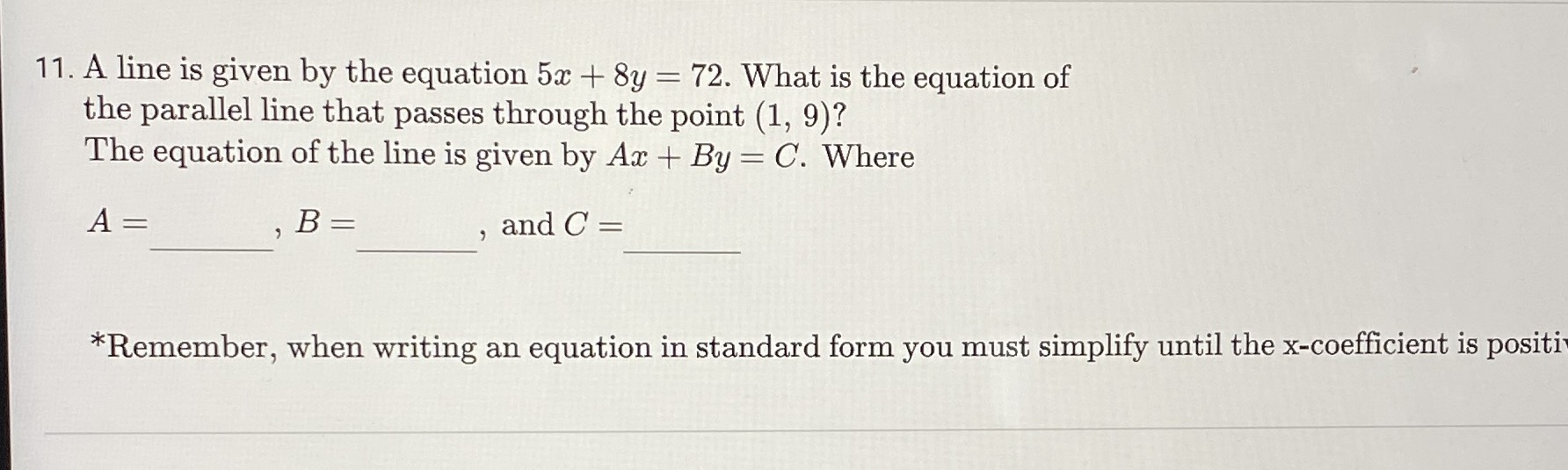### ¿Todavía tienes preguntas de matemáticas?

Pregunte a nuestros tutores expertos
Algebra
Pregunta11. A line is given by the equation $$5 x + 8 y = 72 .$$ What is the equation of the parallel line that passes through the point $$( 1,9 ) ?$$

The equation of the line is given by $$A x + B y = C .$$ Where

$$A = B , B =$$ and $$C =$$

$$*$$ Remember, when writing an equation in standard form you must simplify until the x-coefficient is positi

5x+8y=72

y=9-$$\frac{5}{8}$$x

slope=-5/8

We know that the second line will also have a slope of –5/8, and we are given the point (1,9). We can set up an equation in slope-intercept form and use these values to solve for the y-intercept.

y = mx + b

9 = –5/8(1) + b

9= –5/8 + b

b = 9 + 5/8 = 9.625

Plug the y-intercept back into the equation to get our final answer.

y = (5/8)x + 9.625

Solución
View full explanation on CameraMath App.